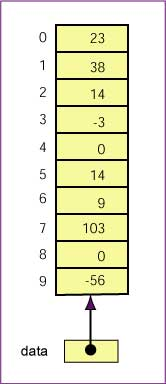Probably not. It would be useful to have an organized way of reading and storing the values.

# Picture of an ArrayAn array is an object that is used to store a list of values.

An array is made out of a contiguous block of memory that is divided into a number of cells. Each cell holds a value, and all the values are of the same type. Sometimes the cells of an array are called slots. In the example array pictured at right, each cell holds an `int`.

The reference variable that points to this array is named `data`. Sometimes the identifier "`data`" is called "the name of the array" although it is really the name of a reference variable.

The cells are indexed 0 through 9. Each cell can be accessed by using its index. For example, `data` is the cell which is indexed by zero (which contains the value 23). `data` is the cell which is indexed by 5 (which contains the value 14).

Facts:

• The cells are numbered sequentially starting at zero.
• If there are N cells in an array, the indexes will be 0 through N-1.
• The length of an array is the number of cells.

Sometimes the index is called a subscript. The expression `data` is usually pronounced "data-sub-five" as if it were an expression from mathematics: `data5`.

The value stored in a cell of an array is sometimes called an element of the array. An array has a fixed number of cells. The values in the cells (the elements) can be changed.

An array is a full-blown object, and consists of more than just its cells. For example, the `length` instance variable holds number of cells of the array. For the above array, `data.length` holds the value 10.

### QUESTION 2:

What value is in `data` ?